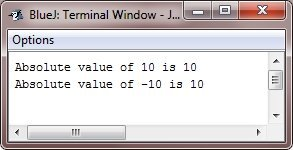# Java ternary or conditional (?:) operator

Java's ternary operator can be used to create if-else statements more quickly. Because it tests a boolean expression and returns one of two potential values depending on the outcome, it is also known as the conditional operator.

## Java ternary (?:) operator syntax

The ternary operator has the following syntax:

`variable = (condition) ? expressionIfTrue : expressionIfFalse;`

If the "condition" evaluates to true, "expressionIfTrue" is assigned to variable; otherwise, "expressionIfFalse" is assigned to variable."

## Java ternary (?:) operator example

Consider the following: Java program as an example demonstrating the ternary operator.

Java Code
```public class JavaProgram {
public static void main(String args[]) {
int a = 100;
int b = 200;
int max = (a > b) ? a : b;
System.out.println("The maximum value is: " + max);
}
}```
Output
`The maximum value is: 200`

The program assigns values to two integer variables, a and b, and then compares those values using the ternary operator. The value of "a" is assigned to the variable "max" if "a" is greater than "b"; otherwise, the value of "b" is assigned to "max." The program then uses the "max" variable to print the maximum value.

The same program can also be written in this way:

```public class JavaProgram {
public static void main(String args[]) {
int a = 100, b = 200;
System.out.println("The maximum value is: " + ((a > b) ? a : b));
}
}```

The following program is another example that demonstrates the ?: operator. It uses the ternary operator to obtain the absolute value of a variable.

```public class JavaProgram {
public static void main(String args[]) {
int i = 10, k;
k = i < 0 ? -i : i;     // get the absolute value of 'i'

System.out.println("Absolute value of " + i + " is " + k);

i = -10;
k = i < 0 ? -i : i;     // get the absolute value of 'i'

System.out.println("Absolute value of " + i + " is " + k);
}
}```

When the above Java program is compiled and executed, it will produce the following output:The same program can also be written using the library function in this way:

```public class JavaProgram {
public static void main(String[] args) {
int i = 10;
int k = Math.abs(i);
System.out.println("Absolute value of " + i + " is " + k);

i = -10;
k = Math.abs(i);
System.out.println("Absolute value of " + i + " is " + k);
}
}```

### Advantages of the ternary operator (?:) in Java

• Concise code: As opposed to if-else statements, the ternary operator enables you to write concise code. This can improve the code's readability and comprehension.
• Reduces the number of lines of code needed: When ternary operators are used instead of if-else statements, fewer lines of code are needed. This may ease management and upkeep of the code.
• Simple to nest: The ternary operator is a flexible tool for challenging programming tasks because it is simple to nest inside other ternary operators or if-else statements.

### Disadvantages of the ternary operator (?:) in Java

• Concise code has advantages, but it can also be difficult to read at times. Coding can be challenging to read and comprehend when using the ternary operator, especially for novices who might not be familiar with the syntax.
• Complex conditions: Because the ternary operator can make the code more difficult to read and maintain, it might not be appropriate for complex conditions.
• Not always appropriate: It's possible that not all circumstances call for the use of the ternary operator. It should only be used when it makes the code simpler to read and comprehend.

Java Online Test

« Previous Tutorial Next Tutorial »